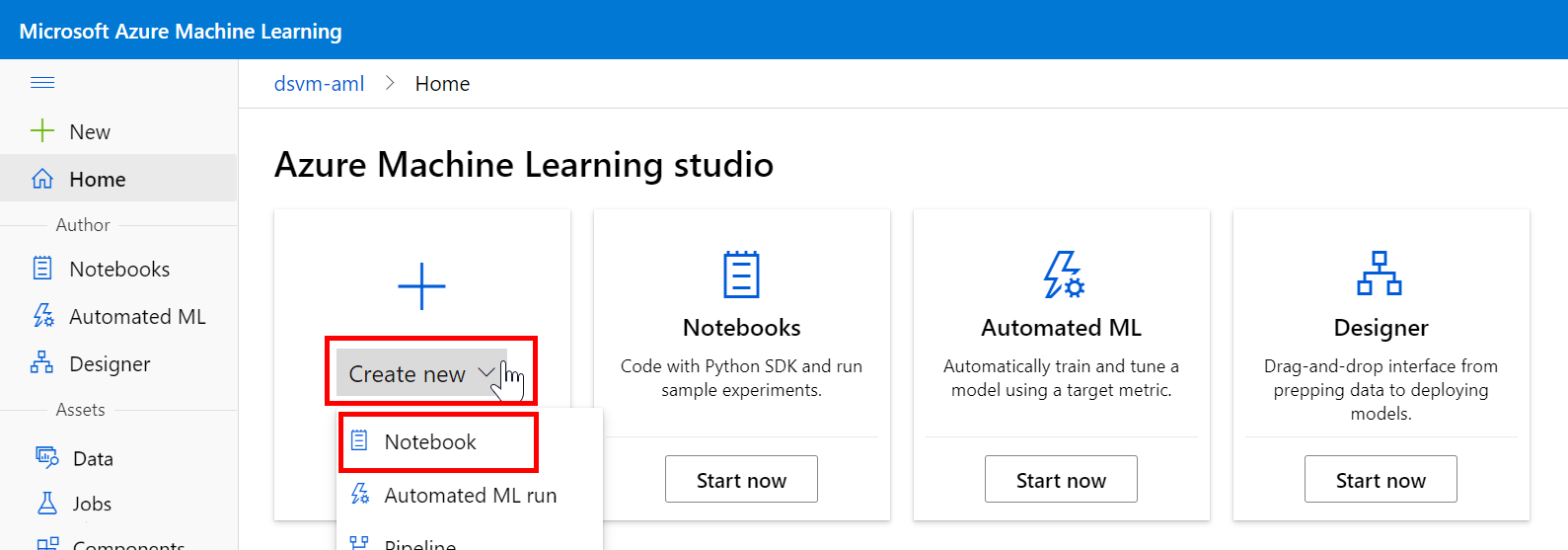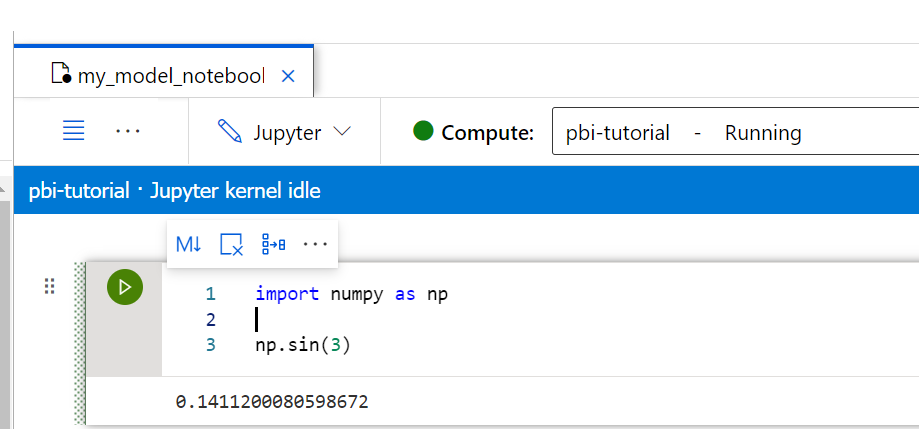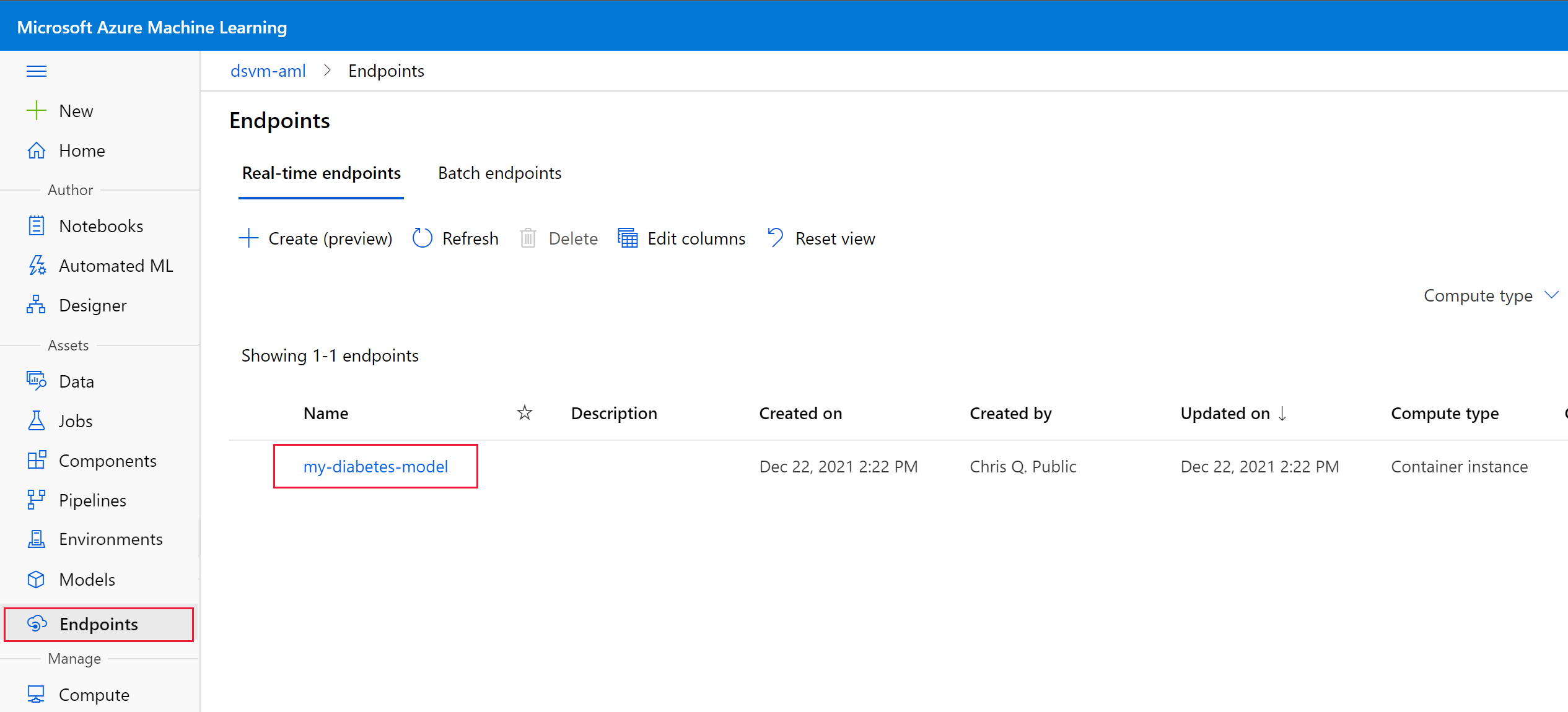# Tutorial: Power BI integration - Create the predictive model with a Jupyter Notebook (part 1 of 2)

APPLIES TO:Python SDK azureml v1

In part 1 of this tutorial, you train and deploy a predictive machine learning model by using code in a Jupyter Notebook. You also create a scoring script to define the input and output schema of the model for integration into Power BI. In part 2, you use the model to predict outcomes in Microsoft Power BI.

In this tutorial, you:

• Create a Jupyter Notebook.
• Create an Azure Machine Learning compute instance.
• Train a regression model by using scikit-learn.
• Write a scoring script that defines the input and output for easy integration into Microsoft Power BI.
• Deploy the model to a real-time scoring endpoint.

## Prerequisites

• An Azure subscription. If you don't already have a subscription, you can use a free trial.
• An Azure Machine Learning workspace. If you don't already have a workspace, see Create workspace resources.
• Introductory knowledge of the Python language and machine learning workflows.

## Create a notebook and compute

On the Azure Machine Learning Studio home page, select Create new > Notebook:On the Create a new file page:

1. Name your notebook (for example, my_model_notebook).
2. Change the File Type to Notebook.
3. Select Create.

Next, to run code cells, create a compute instance and attach it to your notebook. Start by selecting the plus icon at the top of the notebook:On the Create compute instance page:

1. Choose a CPU virtual machine size. For this tutorial, you can choose a Standard_D11_v2, with 2 cores and 14 GB of RAM.
2. Select Next.
3. On the Configure Settings page, provide a valid Compute name. Valid characters are uppercase and lowercase letters, digits, and hyphens (-).
4. Select Create.

In the notebook, you might notice the circle next to Compute turned cyan. This color change indicates that the compute instance is being created:Note

The compute instance can take 2 to 4 minutes to be provisioned.

After the compute is provisioned, you can use the notebook to run code cells. For example, in the cell you can type the following code:

``````import numpy as np

np.sin(3)
``````

Then select Shift + Enter (or select Control + Enter or select the Play button next to the cell). You should see the following output:Now you're ready to build a machine learning model.

## Build a model by using scikit-learn

In this tutorial, you use the Diabetes dataset. This dataset is available in Azure Open Datasets.

### Import data

To import your data, copy the following code and paste it into a new code cell in your notebook.

``````from azureml.opendatasets import Diabetes

diabetes = Diabetes.get_tabular_dataset()
X = diabetes.drop_columns("Y")
y = diabetes.keep_columns("Y")
X_df = X.to_pandas_dataframe()
y_df = y.to_pandas_dataframe()
X_df.info()
``````

The `X_df` pandas data frame contains 10 baseline input variables. These variables include age, sex, body mass index, average blood pressure, and six blood serum measurements. The `y_df` pandas data frame is the target variable. It contains a quantitative measure of disease progression one year after the baseline. The data frame contains 442 records.

### Train the model

Create a new code cell in your notebook. Then copy the following code and paste it into the cell. This code snippet constructs a ridge regression model and serializes the model by using the Python pickle format.

``````import joblib
from sklearn.linear_model import Ridge

model = Ridge().fit(X_df,y_df)
joblib.dump(model, 'sklearn_regression_model.pkl')
``````

### Register the model

In addition to the content of the model file itself, your registered model will store metadata. The metadata includes the model description, tags, and framework information.

Metadata is useful when you're managing and deploying models in your workspace. By using tags, for instance, you can categorize your models and apply filters when you list models in your workspace. Also, if you mark this model with the scikit-learn framework, you'll simplify deploying it as a web service.

Copy the following code and then paste it into a new code cell in your notebook.

``````import sklearn

from azureml.core import Workspace
from azureml.core import Model
from azureml.core.resource_configuration import ResourceConfiguration

ws = Workspace.from_config()

model = Model.register(workspace=ws,
model_name='my-sklearn-model',                # Name of the registered model in your workspace.
model_path='./sklearn_regression_model.pkl',  # Local file to upload and register as a model.
model_framework=Model.Framework.SCIKITLEARN,  # Framework used to create the model.
model_framework_version=sklearn.__version__,  # Version of scikit-learn used to create the model.
sample_input_dataset=X,
sample_output_dataset=y,
resource_configuration=ResourceConfiguration(cpu=2, memory_in_gb=4),
description='Ridge regression model to predict diabetes progression.',
tags={'area': 'diabetes', 'type': 'regression'})

print('Name:', model.name)
print('Version:', model.version)
``````

You can also view the model in Azure Machine Learning Studio. In the menu on the left, select Models:## Define the scoring script

When you deploy a model that will be integrated into Power BI, you need to define a Python scoring script and custom environment. The scoring script contains two functions:

• The `init()` function runs when the service starts. It loads the model (which is automatically downloaded from the model registry) and deserializes it.
• The `run(data)` function runs when a call to the service includes input data that needs to be scored.

Note

The Python decorators in the code below define the schema of the input and output data, which is important for integration into Power BI.

Copy the following code and paste it into a new code cell in your notebook. The following code snippet has cell magic that writes the code to a file named score.py.

``````%%writefile score.py

import json
import pickle
import numpy as np
import pandas as pd
import os
import joblib
from azureml.core.model import Model

from inference_schema.schema_decorators import input_schema, output_schema
from inference_schema.parameter_types.numpy_parameter_type import NumpyParameterType
from inference_schema.parameter_types.pandas_parameter_type import PandasParameterType

def init():
global model
# Replace filename if needed.
path = os.getenv('AZUREML_MODEL_DIR')
model_path = os.path.join(path, 'sklearn_regression_model.pkl')
# Deserialize the model file back into a sklearn model.

input_sample = pd.DataFrame(data=[{
"AGE": 5,
"SEX": 2,
"BMI": 3.1,
"BP": 3.1,
"S1": 3.1,
"S2": 3.1,
"S3": 3.1,
"S4": 3.1,
"S5": 3.1,
"S6": 3.1
}])

# This is an integer type sample. Use the data type that reflects the expected result.
output_sample = np.array()

# To indicate that we support a variable length of data input,
# set enforce_shape=False
@input_schema('data', PandasParameterType(input_sample))
@output_schema(NumpyParameterType(output_sample))
def run(data):
try:
print("input_data....")
print(data.columns)
print(type(data))
result = model.predict(data)
print("result.....")
print(result)
# You can return any data type, as long as it can be serialized by JSON.
return result.tolist()
except Exception as e:
error = str(e)
return error
``````

## Define the custom environment

Next, define the environment to score the model. In the environment, define the Python packages, such as pandas and scikit-learn, that the scoring script (score.py) requires.

To define the environment, copy the following code and paste it into a new code cell in your notebook.

``````from azureml.core.model import InferenceConfig
from azureml.core import Environment

environment = Environment('my-sklearn-environment')
'azureml-defaults',
'inference-schema[numpy-support]',
'joblib',
'numpy',
'pandas',
'scikit-learn=={}'.format(sklearn.__version__)
])

inference_config = InferenceConfig(entry_script='./score.py',environment=environment)
``````

## Deploy the model

To deploy the model, copy the following code and paste it into a new code cell in your notebook:

``````service_name = 'my-diabetes-model'

service = Model.deploy(ws, service_name, [model], inference_config, overwrite=True)
service.wait_for_deployment(show_output=True)
``````

Note

The service can take 2 to 4 minutes to deploy.

If the service deploys successfully, you should see the following output:

``````Tips: You can try get_logs(): https://aka.ms/debugimage#dockerlog or local deployment: https://aka.ms/debugimage#debug-locally to debug if deployment takes longer than 10 minutes.
Running......................................................................................
Succeeded
ACI service creation operation finished, operation "Succeeded"
``````

You can also view the service in Azure Machine Learning Studio. In the menu on the left, select Endpoints:We recommend that you test the web service to ensure it works as expected. To return your notebook, in Azure Machine Learning Studio, in the menu on the left, select Notebooks. Then copy the following code and paste it into a new code cell in your notebook to test the service.

``````import json

The output should look like this JSON structure: `{'predict': [[205.59], [68.84]]}`.